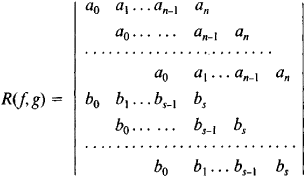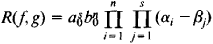# Resultant

Also found in: Dictionary, Thesaurus, Legal, Wikipedia.

## resultant

Maths Physics a single vector that is the vector sum of two or more other vectors

## Resultant

an algebraic expression used to solve systems of algebraic equations. The resultant of the two polynomial equations f(x) = + • • • + a0xn + … + an = 0 and g(x) = b0xs + … + bs = 0 (a0 and b0 may vanish) is the determinantwhere the empty positions are occupied by zeros. The determinant has i rows containing the coefficients a0, a1, …,an and n rows containing the coefficients b0, b1,…. b3, If a0 ≠ 0 and b0 ≠ 0, thenwhere α1, α2, …, αn are the roots of the equation f(x) = 0 and β1, β2, …, βs are the roots of the equation g(x) = 0. The resultant vanishes if, and only if, the two equations have a common root or their leading coefficients are both equal to zero.

Let there be given the two equations P(x,y) = 0 and Q(x,y) = 0, where P and Q are polynomials in x and y. Suppose these polynomials are arranged according to powers of x, and the resultant of the polynomials obtained is equated to zero. An equation in y is then obtained of degree at most sn. Here, η is the degree of P in x and y, and s is the degree of Q. If x = x0, y = y0 is a solution of the given system of equations, then y = y0 is a root of the equation R(f, g) = 0. The process of solving a system of two equations is thereby reduced to the solution of a single equation.

The resultant of a polynomial equation and its derivative is equal, except possibly in sign, to the discriminant of the polynomial equation. If the discriminant is equal to zero, the polynomial equation has multiple roots.

### REFERENCE

Kurosh, A. G. Kurs vysshei algebry, 10th ed. Moscow, 1971.

## Resultant

The resultant of a system of forces is a force equivalent to the given system and equal to the vector sum of the forces: R = ΣFk. A system of forces applied at the same point always has a resultant if R ≠ 0. Any other system of forces applied to a body has a resultant, if R ≠ 0, when the moment of the system either is equal to zero or is perpendicular to R. In this case, the system of forces may be replaced by the resultant of the forces only when the body may be regarded as perfectly rigid. The system may not be replaced when, for example, internal forces are to be determined or when other problems that require the deformation of the body to be taken into account are to be solved. A couple and a system of two forces that are not coplanar are examples of systems of forces that do not have resultants.

## resultant

[ri′zəl·tənt]
(mathematics)
For a set of polynomial equations, a function of the coefficients which equals zero if the equations have at least one solution. Also known as eliminant.
References in periodicals archive ?
Les depots de la clientele ont enregistre, de leur cote, au terme des neufs premiers mois de 2016 un accroissement de 5,0% (+30,601 millions de dinars) par rapport a la meme periode de 2015 resultant essentiellement de l'accroissement des depots a terme (+45,4%), soit +97,999 millions de dinars, ainsi que de l'accroissement des comptes d'epargne qui ont augmente de (+21,6%), soit +9,989 millions de dinars.
The paper discusses a model of AE resultant signal formation during friction wear of CM-based contact surfaces.
Also, the moble robot is successfully takes the resultant path to reach the target position.
It follows that the system of d'Alembert's fictitious forces is a parallel forces system and can be replaced by the resultant vector.
Accordingly, let us consider the following well known classical results, (6)-(8) below, which are involved in the calculation of the Newtonian resultant mass [m.
The antimicrobial activities of the resultant polymer (powder state) was tested against Staphylococcus aureus (S.
The resultant material is either shipped by trailer, as in the case of the fuel chips, or bagged, as in the case of the crumb and fine grind.
For Resultant Fury, a dedicated PA-plans Airman had responsibility for constant coordination among PA, IO, DO, and an information-warfare flight.
Japan has to live with a falling population, but the resultant natural decline in the labor force will tighten the labor market as the economy recovers under structural improvement.
A hexamethylene diisocyanate (HDI) hardener ensures good crosslinking and resistance properties of the resultant coatings, while an isophorone diisocyanate (IPDI) hardener accelerates physical drying.
The resultant forms will, it is anticipated, create new focal elements that will contribute toward the functional and cultural regeneration of the Traiano district of Naples that has suffered isolation and neglect.

Site: Follow: Share:
Open / Close